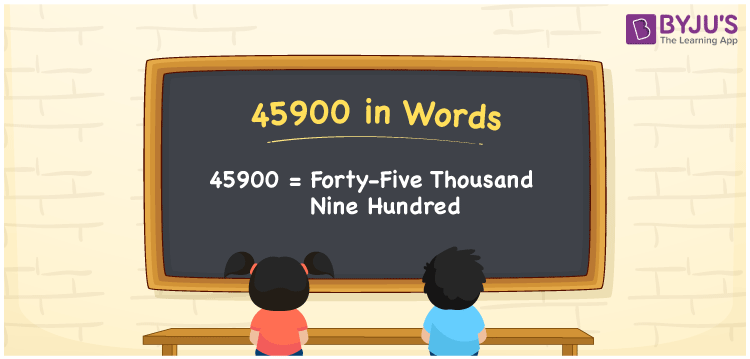# 45900 in Words

45900 in words is written as Forty-five thousand nine hundred. In both the International System of Numerals and the Indian System of Numerals, 45900 in words is written as Forty-five thousand nine hundred. The number 45900 is a Cardinal Number as it represents some quantity. For example, “the population of this city is about 45900”.

 45900 in Words Forty-five thousand nine hundred Forty-five thousand nine hundred in Number 45900

## 45900 in English Words

45900 in English words is read as “Forty-five thousand nine hundred”.## How to Write 45900 in Words?

To write 45900 in words, we shall use the place value chart. In the place value chart, put 4 in the ten thousand, 5 in the thousands, 9 in the hundreds, and 0 in the tens and the ones, respectively. Let us make a place value chart to write the number 45900 in words.

 Ten Thousands Thousands Hundreds Tens Ones 4 5 9 0 0

Thus, we can write the expanded form as

4 × Ten Thousand + 5 × Thousand + 9 × Hundred + 0 × Ten + 0 × One

= 4 × 10000 + 5 × 1000 + 9 × 100 + 0 × 10 + 0 × 1

= 40000 + 5000 + 900 + 0 + 0

= 45900

= Forty-five thousand nine hundred.

45900 is a natural number that lies between 45899 and 45901.

45900 in words – Forty-five thousand nine hundred

• Is 45900 an odd number? – No
• Is 45900 an even number? – Yes
• Is 45900 a perfect square number? – No
• Is 45900 a perfect cube number? – No
• Is 45900 a prime number? – No
• Is 45900 a composite number? – Yes

## Frequently Asked Questions on 45900 in Words

Q1

### How to write 45900 in words?

45900 in words is written as Forty-five thousand nine hundred.
Q2

### How to write 45900 in words in the International and Indian System of Numerals?

In both, the system of numerals, 45900 in words, is written as Forty-five thousand nine hundred.
Q3

### How to write 45900 in a place value chart?

In the place value chart, write 4 in the ten thousand, 5 in the thousands, 9 in the hundreds, and 0 in the tens and ones, respectively.